# Behavior laws and constitutive equations: definition

## Introduction

The solving of an electromagnetic problem consists of solving a set of equations:

• the Maxwell equations, which represent the backbone of the theory
• the constitutive equations of the matter, which model the material properties.

The Maxwell equations are presented in the chapters pertaining to magnetic and electric applications: Magnetic applications: principles and Electric applications: principes.

This chapter will cover the constitutive equations of the matter.

## Constitutive equations

The constitutive equations of the matter characterize the different materials: conductive, magnetic, dielectric or thermal (by thermal conductivity or volumetric heat capacity) .

They are presented in the table below.

Material Constitutive equation Material property
magnetic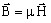(1) μ: permeability H/m
dielectric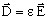(2) ε: permittivity F/m
conductive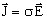(3) σ: conductivity Ω-1.m-1
thermal (thermal conductivity)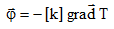(4) k: tensor of the thermal conductivity W/m/degree
thermal (volumetric heat capacity)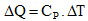(5) ρCp (T): volumetric heat capacity (J/m3/degree)

## Denomination

These constitutive equations express:

• (1) law of magnetic behavior
• (2) law of dielectric behavior
• (3) law of electric behavior or local formulation of Ohm's law
• (4) law of thermal behavior by conductivity
• (5) law of thermal behavior by volumetric heat capacity